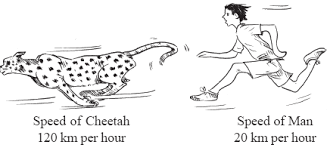1. /
2. CBSE
3. /
4. Class 07
5. /
6. Comparing Quantities worksheet for...

# Comparing Quantities worksheet for class 7### myCBSEguide App

Download the app to get CBSE Sample Papers 2023-24, NCERT Solutions (Revised), Most Important Questions, Previous Year Question Bank, Mock Tests, and Detailed Notes.

## Comparing Quantities worksheet for class 7 Important Topics

• Comparing Quantities
• Equivalent Ratios
• Percentage : Another way of comparing quantities
• Converting fractional and decimal numbers to percentage
• Converting percentage to fractions or decimal numbers
• Uses of Percentage : Interpreting and converting percentages
• Uses of Percentage : Ratio to Percent, Increase of Decrease as percent
• Profit or Loss as Percentage
• Introduction to simple interest for one and multiple year## Some important Facts about Comparing Quantities worksheet for class 7

1. We are often required to compare two quantities in our daily life. They may be heights, weights, salaries, marks etc.
2. While comparing heights of two persons with heights150 cm and 75 cm, we write it as the ratio 150 : 75 or 2 : 1.
3. Two ratios can be compared by converting them to like fractions. If the two fractions are equal, we say the two given ratios are equivalent.
4. If two ratios are equivalent then the four quantities are said to be in proportion. For example, the ratios 8 : 2 and 16 : 4 are equivalent therefore 8, 2, 16 and 4 are in proportion.
5. A way of comparing quantities is percentage. Percentages are numerators of fractions with denominator 100. Per cent means per hundred.

## NCERT class 7Mathematics Solved Worksheets

• Chapter 1 – Integers
• Chapter 2 – Fractions and Decimals
• Chapter 3 – Data Handling
• Chapter 4 – Simple Equations
• Chapter 5 – Lines and Angles
• Chapter 6 – Practical Geometry
• Chapter 7 – The Triangle and its Properties
• Chapter 8 – Congruence of triangles
• Chapter 9 – Comparing Quantities
• Chapter 10 – Rational numbers
• Chapter 11 – Perimeter and Area
• Chapter 12 – Algebraic Expressions
• Chapter 13 – Exponents and Powers
• Chapter 14 – Symmetry
• Chapter 15 – Visualizing Solid Shapes

## CHARGE GIVEN ON BORROWED MONEY OR SIMPLE INTERESTS

Sohini said that they were going to buy a new scooter. Mohan asked her whether they had the money to buy it. Sohini said her father was going to take a loan from a bank. The money you borrow is known as sum borrowed or principal.

This money would be used by the borrower for some time before it is returned. For keeping this money for some time the borrower has to pay some extra money to the bank. This is known as Interest.

You can find the amount you have to pay at the end of the year by adding the sum borrowed and the interest. That is, Amount = Principal + Interest.

Interest is generally given in per cent for a period of one year. It is written as say 10% per year or per annum or in short as 10% p.a. (per annum). 10% p.a. means on every  100 borrowed,  10 is the interest you have to pay for one year. Let us take an example and see how this works.

To download Printable worksheets for class 7 Mathematics and Science; do check myCBSEguide app or website. myCBSEguide provides sample papers with solution, test papers for chapter-wise practice, NCERT solutions, NCERT Exemplar solutions, quick revision notes for ready reference, CBSE guess papers and CBSE important question papers. Sample Paper all are made available through the best app for CBSE students and myCBSEguide website.Test Generator

Create question paper PDF and online tests with your own name & logo in minutes.myCBSEguide

Question Bank, Mock Tests, Exam Papers, NCERT Solutions, Sample Papers, Notes